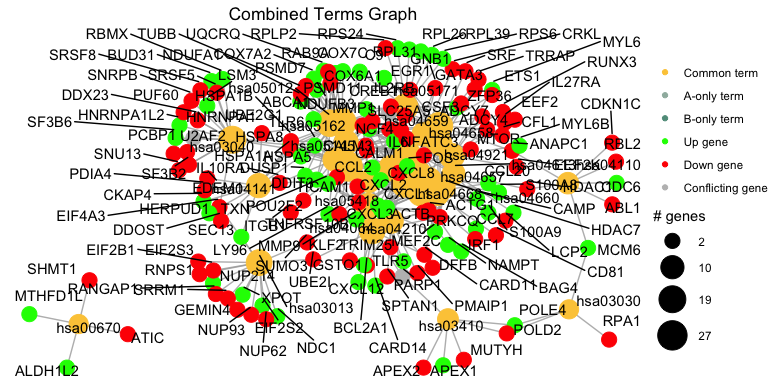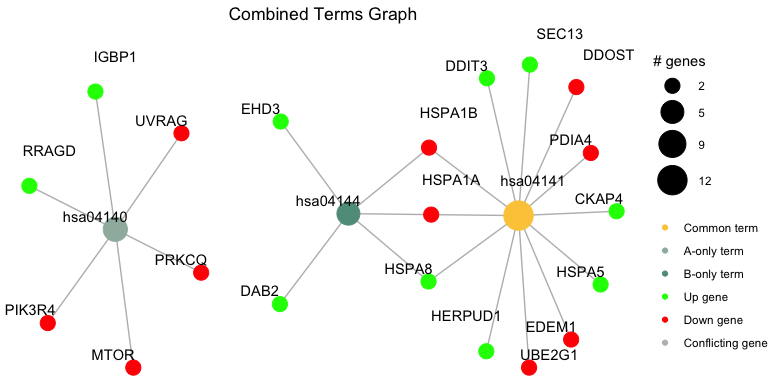# Comparing Two pathfindR Results

The function combine_pathfindR_results() allows combination of two pathfindR active-subnetwork-oriented enrichment analysis results for investigating common and distinct terms between the groups. Below is an example for comparing results using two different rheumatoid arthritis-related data sets (RA_output and RA_comparison_output).

combined_df <- combine_pathfindR_results(result_A = RA_output,
result_B = RA_comparison_output,
plot_common = FALSE)
#> You may run combined_results_graph() to create visualizations of combined term-gene graphs of selected terms

By default, combine_pathfindR_results() plots the term-gene graph for the common terms in the combined results. For not plotting the graph, set plot_common = FALSE.

The function combined_results_graph() can be used to create this graph (using only selected terms etc.) later on. By default, the function creates the graph using all common terms:

combined_results_graph(combined_df)
#> Warning: ggrepel: 19 unlabeled data points (too many overlaps). Consider
#> increasing max.overlapsBy supplying a vector of selected terms to the selected_terms arguments, you may plot the term-gene graph for the selected terms:

combined_results_graph(combined_df,
selected_terms = c("hsa04144", "hsa04141", "hsa04140"))By default, combined_results_graph() creates the graph using term IDs. To use term descriptions instead, set use_description = TRUE:

combined_results_graph(combined_df,
use_description = TRUE,
selected_terms = combined_df\$Term_Description[1:4])

For changing the layout of the graph ("auto" by default), you may use the layout argument.

For changing how the sizes of the term nodes are determined, you may use the node_size argument. The options are "num_genes" (default) and "p_val" for using the number of significant genes in the term and the -log10(p) value of the term, respectively:

combined_results_graph(combined_df,
selected_terms = c("hsa04144", "hsa04141", "hsa04140"),
node_size = "p_val")